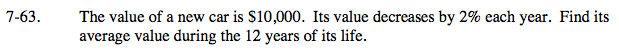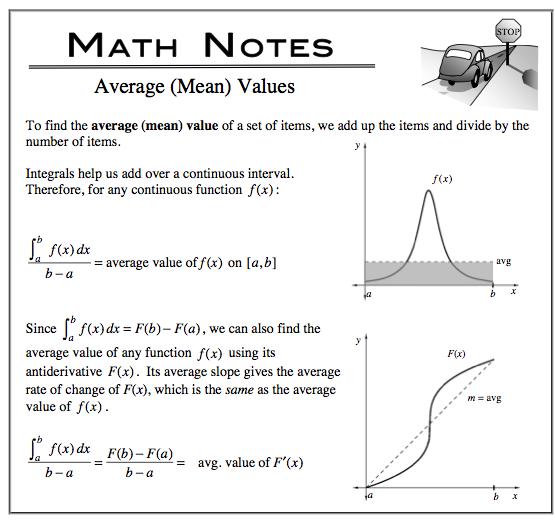### Home > CALC > Chapter 7 > Lesson 7.2.1 > Problem7-63

7-63.
1. The value of a new car is \$10,000. Its value decreases by 2% each year. Find its average value during the 12 years of its life. Homework Help ✎

2.Find the average value of an exponential equation.

$V_{\text{avg}}=\frac{1}{12}\int_0^{12}V(t)dt$